Generalization: Model Validation¶

Data Science School, Nyeri, Kenya¶

16th June 2015 Neil Lawrence¶

If we had to summarise the objectives of machine learning in one word, a very good candidate for that word would be generalization. What is generalization? From a human perspective it might be summarised as the ability to take lessons learned in one domain and apply them to another domain. If we accept the definition given in the first session for machine learning, $$\text{data} + \text{model} \rightarrow \text{prediction}$$ then we see that without a model we can't generalise: we only have data. Data is fine for answering very specific questions, like "Who won the Olympic Marathon in 2012?", because we have that answer stored, however, we are not given the answer to many other questions. For example, Alan Turing was a formidable marathon runner, in 1946 he ran a time 2 hours 46 minutes (just under four minutes per kilometer, faster than I and most of the other Endcliffe Park Run runners can do 5 km). What is the probability he would have won an Olympics if one had been held in 1946?*Alan Turing, in 1946 he was only 11 minutes slower than the winner of the 1948 games. Would he have won a hypothetical games held in 1946? Source: [Alan Turing Internet Scrapbook](http://www.turing.org.uk/scrapbook/run.html).*
To answer this question we need to generalize, but before we formalize the concept of generalization let's introduce some formal representation of what it means to generalize in machine learning.

Expected Loss¶

Our objective function so far has been the negative log likelihood, which we have minimized (via the sum of squares error) to obtain our model. However, there is an alternative perspective on an objective function, that of a loss function. A loss function is a cost function associated with the penalty you might need to pay for a particular incorrect decision. One approach to machine learning involves specifying a loss function and considering how much a particular model is likely to cost us across its lifetime. We can represent this with an expectation. If our loss function is given as $L(y, x, \mathbf{w})$ for a particular model that predicts $y$ given $x$ and $w$ then we are interested in minimizing the expected loss under the likely distribution of $y$ and $x$. To understand this formally we define the true distribution of the data samples, $y$, $x$. This is a very special distribution that we don't have access to very often, and to represent that we define it with a special letter 'P', $\mathbb{P}(y, x)$. If we genuinely pay $L(y, x, \mathbf{w})$ for every mistake we make, and the future test data is genuinely drawn from $\mathbb{P}(y, x)$ then we can define our expected loss, or risk, to be, $$R(\mathbf{w}) = \int L(y, x, w) \mathbb{P}(y, x) \text{d}y \text{d}x.$$ Of course, in practice, this value can't be computed but it serves as a reminder of what it is we are aiming to minimize and under certain circumstances it can be approximated.

Sample Based Approximations¶

A sample based approximation to an expectation involves replacing the true expectation with a sum over samples from the distribution. $$\int f(z) p(z) \text{d}y \text{d}z\approx \frac{1}{s}\sum_{i=1}^s f(z_i).$$ if $\{z_i\}_{i=1}^s$ are a set of $s$ independent and identically distributed samples from the distribution $p(z)$. This approximation becomes better for larger $s$, although the rate of convergence to the true integral will be very dependent on the distribution $p(z)$ and the function $f(z)$.

That said, this means we can approximate our true integral with the sum, $$R(\mathbf{w}) \approx \frac{1}{n}\sum_{i=1}^n L(y_i, x_i, w),$$ if $y_i$ and $x_i$ are independent samples from the true distribution $\mathbb{P}(y, x)$. Minimizing this sum directly is known as empirical risk minimization. The sum of squares error we have been using can be recovered for this case by considering a squared loss, $$L(y, x, \mathbf{w}) = (y-\mathbf{w}^\top\boldsymbol{\phi}(x))^2$$ which gives an empirical risk of the form $$R(\mathbf{w}) \approx \frac{1}{n} \sum_{i=1}^n (y_i - \mathbf{w}^\top \boldsymbol{\phi}(x_i))^2$$ which up to the constant $\frac{1}{n}$ is identical to the objective function we have been using so far.

Estimating Risk through Validation¶

Unfortuantely, minimising the empirial risk only guarantees something about our performance on the training data. If we don't have enough data for the approximation to the risk to be valid, then we can end up performing significantly worse on test data. Fortunately, we can also estimate the risk for test data through estimating the risk for unseen data.

The main trick here is to 'hold out' a portion of our data from training and use the models performance on that sub-set of the data as a proxy for the true risk. This data is known as 'validation' data. It contrasts with test data, because it's values are known at the model design time. However, in contrast to test date we don't use it to fit our model. This means that it doesn't exhibit the same bias that the empirical risk does when estimating the true risk.

In this lab we will explore techniques for model selection that make use of validation data. Data that isn't seen by the model in the learning (or fitting) phase, but is used to validate our choice of model from amoungst the different designs we have selected.

In machine learning, we are looking to minimise the value of our objective function $E$ with respect to its parameters $\mathbf{w}$. We do this by considering our training data. We minimize the value of the objective function as it's observed at each training point. However we are really interested in how the model will perform on future data. For evaluating that we choose to hold out a portion of the data for evaluating the quality of the model.

We will review the different methods of model selection on the Olympics marathon data. Firstly we import the olympics data.

In :
import numpy as np
import pods
data = pods.datasets.olympic_marathon_men()
x = data['X']
y = data['Y']
Acquiring resource: olympic_marathon_men

Details of data:
Olympic mens' marathon gold medal winning times from 1896 to 2012. Time given in pace (minutes per kilometer). Data is originally downloaded and collated from Wikipedia, we are not responsible for errors in the data

Data will be stored in /home/lionfish/ods_data_cache/olympic_marathon_men.

yes
olympicMarathonTimes.csv
[==============================]   0.001/0.001MB

We can plot them to check that they've loaded in correctly.

In :
%matplotlib inline
import pylab as plt
plt.plot(x, y, 'rx')
Out:
[<matplotlib.lines.Line2D at 0x7f33240e1650>]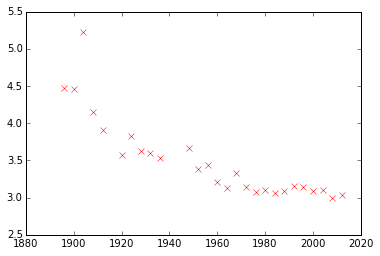Hold Out Validation¶

The first thing we'll do is fit a standard linear model to the data. We recall from previous lectures and lab classes that to do this we need to solve the system $$\boldsymbol{\Phi}^\top \boldsymbol{\Phi} \mathbf{w} = \boldsymbol{\Phi}^\top \mathbf{y}$$ for $\mathbf{w}$ and use the resulting vector to make predictions at the training points and test points, $$\mathbf{f} = \boldsymbol{\Phi} \mathbf{w}.$$ The prediction function can be used to compute the objective function, $$E(\mathbf{w}) = \sum_{i}^n (y_i - \mathbf{w}^\top\phi(\mathbf{x}_i))^2$$ by substituting in the prediction in vector form we have $$E(\mathbf{w}) = (\mathbf{y} - \mathbf{f})^\top(\mathbf{y} - \mathbf{f})$$

To build our model, first we will create a python function that computes $\boldsymbol{\Phi}$ for the linear basis, $$\boldsymbol{\Phi} = \begin{bmatrix} \mathbf{x} & \mathbf{1}\end{bmatrix}$$ We've named the function linear. Phi should be in the form of a design matrix and x should be in the form of a numpy two dimensional array with $n$ rows and 1 column So calls to the function are in the following form:

Phi = linear(x)

We create a python function that accepts, as arguments, a python function that defines a basis (like the one you've just created called linear) as well as a set of inputs and a vector of parameters. Your new python function should return a prediction. Name your function prediction. The return value f should be a two dimensional numpy array with $n$ rows and $1$ column, where $n$ is the number of data points. Calls to your function should be in the following form:

f = prediction(w, x, linear)

We also create a python function that computes the sum of squares objective function (or error function). It should accept your input data (or covariates) and target data (or response variables) and your parameter vector w as arguments. It should also accept a python function that represents the basis. Calls to your function should be in the following form:

e = objective(w, x, y, linear)

Finally we create a function that solves the linear system for the set of parameters that minimizes the sum of squares objective. It should accept input data, target data and a python function for the basis as the inputs. Calls to your function should be in the following form:

w = fit(x, y, linear)

Let's fit a linear model to the olympic data using these functions and plot the resulting prediction between 1890 and 2020. Set the title of the plot to be the error of the fit on the training data.

In :
def linear(x):
"Linear basis function computation"
return np.hstack([x, np.ones_like(x)])

def prediction(w, x, basis):
"Compute the basis functions"
Phi = basis(x)
return np.dot(Phi, w)

def objective(w, x, y, basis):
"Compute the objective function"
f = prediction(w, x, basis)
# OR:  return np.dot((y-f).T, (y-f))
return np.sum((y-f)**2)

def fit(x, y, basis):
"Obtain the model parameters for the model with the lowest error."
Phi = basis(x)
return np.linalg.solve(np.dot(Phi.T, Phi), np.dot(Phi.T, y))

def fit_and_plot(x, y, basis):
w = fit(x, y, basis)
x_pred = np.linspace(1890, 2020, 100)[:, None]
f_pred = prediction(w, x_pred, basis)
import pylab as plt
plt.plot(x_pred, f_pred)
plt.plot(x, y, 'rx')
plt.title('Error: ' + str(objective(w, x, y, basis)))

fit_and_plot(x, y, linear)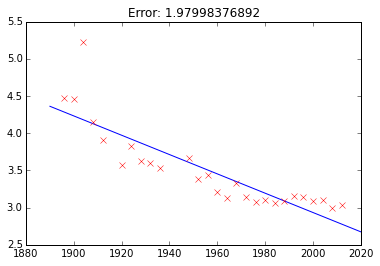Polynomial Fit: Training Error¶

The next thing we'll do is consider a quadratic fit. We will compute the training error for the two fits.

Question 2¶

In this question we extend the code above to a non-linear basis (a quadratic function).

Start by creating a python-function called quadratic. It should compute the quadratic basis. $$\boldsymbol{\Phi} = \begin{bmatrix} \mathbf{1} & \mathbf{x} & \mathbf{x}^2\end{bmatrix}$$ It should be called in the following form:

Use this to compute the quadratic fit for the model, again plotting the result titled by the error.

In :
return np.hstack((np.ones_like(x), x, x**2))

def fit_and_plot(x, y, basis):
w = fit(x, y, basis)
x_pred = np.linspace(1890, 2020, 100)[:, None]
f_pred = prediction(w, x_pred, basis)
import pylab as plt
plt.plot(x_pred, f_pred)
plt.plot(x, y, 'rx')
plt.title('Error: ' + str(objective(w, x, y, basis)))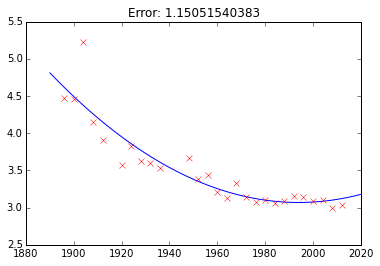Hold Out Data¶

You have a conclusion as to which model fits best under the training error, but how do the two models perform in terms of validation? In this section we consider hold out validation. In hold out validation we remove a portion of the training data for validating the model on. The remaining data is used for fitting the model (training). Because this is a time series prediction, it makes sense for us to hold out data at the end of the time series. This means that we are validating on future predictions. We will hold out data from after 1980 and fit the model to the data before 1980.

In :
# select indices of data to 'hold out'
indices_hold_out = np.flatnonzero(x>1980)

# Create a training set
x_train = np.delete(x, indices_hold_out, axis=0)
y_train = np.delete(y, indices_hold_out, axis=0)

# Create a hold out set
x_valid = np.take(x, indices_hold_out, axis=0)
y_valid = np.take(y, indices_hold_out, axis=0)

Question 3¶

For both the linear and quadratic models, fit the model to the data up until 1980 and then compute the error on the held out data (from 1980 onwards). Which model performs better on the validation data?

In :
def fit_and_plot_valid(x, y, x_valid, y_valid, basis):
w = fit(x, y, basis)
x_pred = np.linspace(1890, 2020, 100)[:, None]
f_pred = prediction(w, x_pred, basis)
import pylab as plt
plt.plot(x_pred, f_pred)
plt.plot(x, y, 'rx')
plt.plot(x_valid, y_valid, 'bo')
print 'Error for', basis.__name__, 'basis', objective(w, x_valid, y_valid, basis)

fit_and_plot_valid(x_train, y_train, x_valid, y_valid, linear)
Error for linear basis 1.91159725287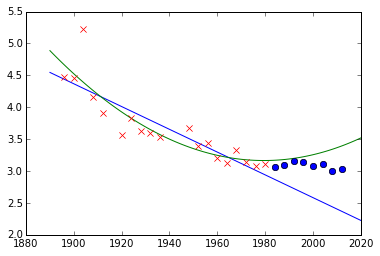Richer Basis Set¶

Now we have an approach for deciding which model to retain, we can consider the entire family of polynomial bases, with arbitrary degrees.

Question 4¶

Now we are going to build a more sophisticated form of basis function, one that can accept arguments to its inputs (similar to those we used in this lab). Here we will start with a polynomial basis.

def polynomial(x, degree, loc, scale):
degrees = np.arange(degree+1)
return ((x-loc)/scale)**degrees

The basis as we've defined it has three arguments as well as the input. The degree of the polynomial, the scale of the polynomial and the offset. These arguments need to be passed to the basis functions whenever they are called. Modify your code to pass these additional arguments to the python function for creating the basis. Do this for each of your functions predict, fit and objective. You will find *args (or **kwargs) useful.

Write code that tries to fit different models to the data with polynomial basis. Use a maximum degree for your basis from 0 to 17. For each polynomial store the hold out validation error and the training error. When you have finished the computation plot the hold out error for your models and the training error for your p. When computing your polynomial basis use offset=1956. and scale=120. to ensure that the data is mapped (roughly) to the -1, 1 range.

Which polynomial has the minimum training error? Which polynomial has the minimum validation error?

In :
def polynomial(x, degree, loc, scale):
degrees = np.arange(degree)
return ((x-loc)/scale)**degrees

def prediction(w, x, basis, **kwargs):
"Compute the basis functions"
Phi = basis(x, **kwargs)
return np.dot(Phi, w)

def fit(x, y, basis, **kwargs):
"Obtain the model parameters for the model with the lowest error."
Phi = basis(x, **kwargs)
return np.linalg.solve(np.dot(Phi.T, Phi), np.dot(Phi.T, y))

def objective(w, x, y, basis, **kwargs):
"Compute the objective function"
f = prediction(w, x, basis, **kwargs)
return np.sum((y-f)**2)

def fit_and_valid(x, y, x_valid, y_valid, basis, **kwargs):
w = fit(x, y, basis, **kwargs)
x_pred = np.linspace(1890, 2020, 100)[:, None]
f_pred = prediction(w, x_pred, basis, **kwargs)
return objective(w, x_valid, y_valid, basis, **kwargs), objective(w, x_train, y_train, basis, **kwargs)
In :
loc = 1956.
scale = 120.
max_degree = 17
degrees = np.arange(max_degree+1)
train_err = np.zeros(max_degree+1)
valid_err = np.zeros(max_degree+1)
for i, degree in enumerate(degrees):
valid_err[i], train_err[i] = fit_and_valid(x_train, y_train, x_valid, y_valid,
basis=polynomial, degree=degree, loc=loc, scale=scale)

import matplotlib.pyplot as plt
plt.semilogy(degrees, valid_err, 'r')
plt.semilogy(degrees, train_err, 'b')
plt.xlabel('degree')
plt.ylabel('error')

plt.figure()
plt.plot(degrees, valid_err, 'rx-')
ax = plt.gca() # a handle to get the current axis
ax.set_yscale('log')
plt.title('Validation error')
plt.xlabel('Polynomial order')
plt.ylabel('Validation Error')
print 'Validation error is:',valid_err

plt.figure()
plt.plot(degrees, train_err, 'bx-')
ax = plt.gca() # a handle to get the current axis
#ax.set_yscale('log')
plt.title('Training error')
plt.xlabel('Polynomial order')
plt.ylabel('Error')
print 'Training error is:',train_err
Validation error is: [  7.61787797e+01   2.81331280e+00   1.91159725e+00   3.37505824e-01
2.51374553e+00   2.63705749e+01   8.45275311e+02   4.48628466e+03
7.64335184e+02   1.16058247e+04   5.21645468e+05   1.40450542e+08
4.05079801e+08   7.18912824e+08   7.38764438e+10   1.25368864e+11
1.98436018e+13   1.68542331e+11]
Training error is: [  2.62529979e+02   5.73633101e+00   1.39277676e+00   1.09853619e+00
1.04391283e+00   1.02421204e+00   8.10242329e-01   6.28719142e-01
6.10287178e-01   6.08856464e-01   5.97169122e-01   3.18721861e-01
1.96608448e-01   1.74204960e-01   1.29463118e-01   1.11050053e-01
3.67259819e-02   2.94187632e-02]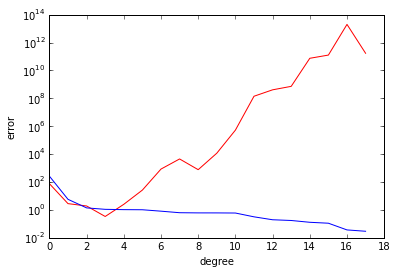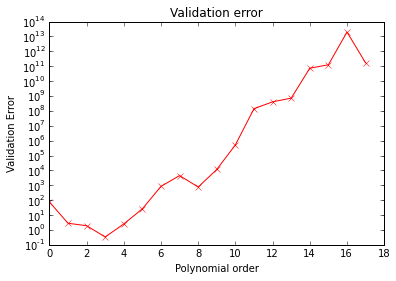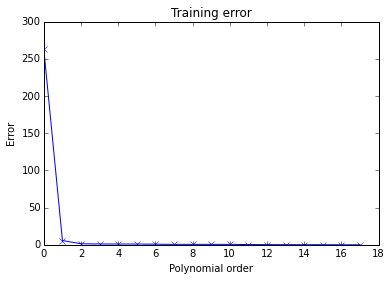Leave One Out Validation¶

Hold out validation uses a portion of the data to hold out and a portion of the data to train on. There is always a compromise between how much data to hold out and how much data to train on. The more data you hold out, the better the estimate of your performance at 'run-time' (when the model is used to make predictions in real applications). However, by holding out more data, you leave less data to train on, so you have a better validation, but a poorer quality model fit than you could have had if you'd used all the data for training. Leave one out cross validation leaves as much data in the training phase as possible: you only take one point out for your validation set. However, if you do this for hold-out validation, then the quality of your validation error is very poor because you are testing the model quality on one point only. In cross validation the approach is to improve this estimate by doing more than one model fit. In leave one out cross validation you fit $n$ different models, where $n$ is the number of your data. For each model fit you take out one data point, and train the model on the remaining $n-1$ data points. You validate the model on the data point you've held out, but you do this $n$ times, once for each different model. You then take the average of all the $n$ badly estimated hold out validation errors. The average of this estimate is a good estimate of performance of those models on the test data.

Question 5¶

Write code that computes the leave one out validation error for the olympic data and the polynomial basis. Use the functions you have created above: objective, fit, polynomial. Compute the leave-one-out cross validation error for basis functions containing a maximum degree from 0 to 17.

In :
def leave_out(x, y, indices):
# Create a training set
x_train = np.delete(x, indices, axis=0)
y_train = np.delete(y, indices, axis=0)

# Create a hold out set
x_valid = np.take(x, indices, axis=0)
y_valid = np.take(y, indices, axis=0)

return x_train, y_train, x_valid, y_valid

max_degree = 17
valid_err = np.zeros(max_degree+1)
degrees = np.arange(max_degree+1)

for i, degree in enumerate(degrees):
for j in xrange(x.shape):
x_train, y_train, x_valid, y_valid = leave_out(x, y, j)# select indices of data to 'hold out'
ve,tr_err= fit_and_valid(x_train, y_train, x_valid, y_valid, polynomial, degree=degree, loc=loc, scale=scale)
valid_err[i] += ve
valid_err[i]/=x.shape
print i,valid_err[i]
print "Leave one out cross validation chooses polynomial of degree", degrees[np.argmin(valid_err)]

import matplotlib.pyplot as plt
plt.semilogy(degrees, valid_err)
plt.xlabel('degree')
_ = plt.ylabel('validation error')
0 12.5447688268
1 0.308421858191
2 0.090600708168
3 0.0579499239453
4 0.0598070506847
5 0.0692741875247
6 0.0883611058708
7 0.0819283019203
8 0.0581151338661
9 0.122602157488
10 0.371787926486
11 1.0449075849
12 2.56440465504
13 5.45939047257
14 8.31901072149
15 5.05525664947
16 31.7922778679
17 111.926397735
Leave one out cross validation chooses polynomial of degree 3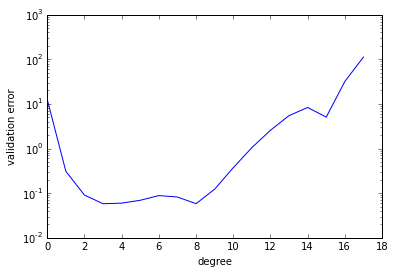$k$-fold Cross Validation¶

Leave one out cross validation produces a very good estimate of the performance at test time, and is particularly useful if you don't have a lot of data. In these cases you need to make as much use of your data for model fitting as possible, and having a large hold out data set (to validate model performance) can have a significant effect on the size of the data set you have to fit your model, and correspondingly, the complexity of the model you can fit. However, leave one out cross validation involves fitting $n$ models, where $n$ is your number of training data. For the olympics example, this is only 27 model fits, but in practice many data sets consist thousands or millions of data points, and fitting many millions of models for estimating validation error isn't really practical. One option is to return to hold out validation, but another approach is to perform $k$-fold cross validation. In $k$-fold cross validation you split your data into $k$ parts. Then you use $k-1$ of those parts for training, and hold out one part for validation. Just like we did for the hold out validation above. In cross validation, however, you repeat this process. You swap the part of the data you just used for validation back in to the training set and select another part for validation. You then fit the model to the new training data and validate on the portion of data you've just extracted. Each split of training/validation data is called a fold and since you do this process $k$ times, the procedure is known as $k$-fold cross validation. The term cross refers to the fact that you cross over your validation portion back into the training data every time you perform a fold.

Question 6¶

Perform $k$-fold cross validation on the olympic data with your polynomial basis. Use $k$ set to 5 (e.g. five fold cross validation). Do the different forms of validation select different models? Does five fold cross validation always select the same model?

Note: The data doesn't divide into 5 equal size partitions for the five fold cross validation error. Don't worry about this too much. Two of the partitions will have an extra data point. You might find np.random.permutation? useful.

In :
parts = 5
max_degree = 17
# randomize the order of the indices to leave out
indices = np.random.permutation(x.shape)
# define partition boundaries on the random order
boundary = np.around(np.linspace(0, x.shape, parts+1))
valid_err = np.zeros(max_degree+1)
degrees = np.arange(max_degree+1)
for i, degree in enumerate(degrees):
for part in xrange(parts):
# leave out the following part
part_indices = indices[boundary[part]:boundary[part+1]]
x_train, y_train, x_valid, y_valid = leave_out(x, y, part_indices)
# compute validation error
ve, tmp = fit_and_valid(x_train, y_train, x_valid, y_valid, polynomial, degree=degree, loc=loc, scale=scale)
valid_err[i] += ve
valid_err[i]/=x.shape
print valid_err[i]
print parts, "fold cross validation chooses polynomial of degree", degrees[np.argmin(valid_err)]

import matplotlib.pyplot as plt
plt.semilogy(degrees, valid_err)
plt.xlabel('degree')
_ = plt.ylabel('validation error')
12.5447688268
0.350038954399
0.103149714301
0.0722729791484
0.0704312050739
0.0729864335142
0.10135915918
0.128295604088
0.115221704885
0.123418393142
0.483768969935
2.87870915215
8.74648074676
114.876657222
196.867887494
76.6644851963
517.284734382
64340.2484154
5 fold cross validation chooses polynomial of degree 4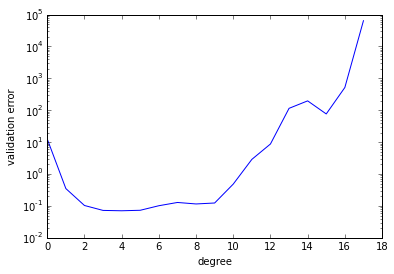Bias Variance Dilemma¶

(quickly mention in lecture)